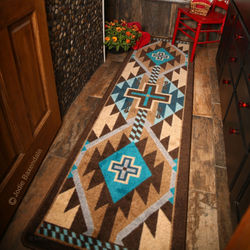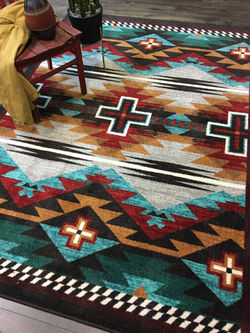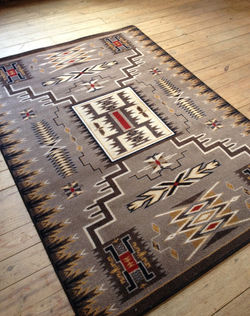## **Please note: rugs are made to order so are not returnable.## Whisky River - Turquoise

### Sizes and prices as follows: 3x4= \$124.95, 4x5= \$189.95, 2x8= \$199.95, 5x8= \$369.95, 8x11=\$699.95, 8' round-\$699.95, 11x13= \$1199.95## Rustic Cross - Sunset

### Sizes and prices as follows: 3x4= \$124.95, 4x5= \$189.95, 2x8= \$199.95, 5x8= \$369.95, 8x11=\$699.95, 8' round-\$699.95## Rustic Cross - Turquoise

### Sizes and prices as follows: 3x4= \$124.95, 4x5= \$189.95, 2x8= \$199.95, 5x8= \$369.95, 8x11=\$699.95, 8' round-\$699.95## Grand River - Black

### Sizes and prices as follows: 3x4= \$124.95, 4x5= \$189.95, 2x8= \$199.95, 5x8= \$369.95, 8x11=\$699.95, 8' round-\$699.95### Sizes and prices as follows: 3x4= \$124.95, 4x5= \$189.95, 2x8= \$199.95, 5x8= \$369.95, 8x11=\$699.95, 8' round-\$699.95## Double Cross - Gray

### Sizes and prices as follows: 3x4= \$124.95, 4x5= \$189.95, 2x8= \$199.95, 5x8= \$369.95, 8x11=\$699.95, 8' round-\$699.95## Whisky River - Rust

### Sizes and prices as follows: 3x4= \$124.95, 4x5= \$189.95, 2x8= \$199.95, 5x8= \$369.95, 8x11=\$699.95, 8' round-\$699.95## Bow Strings - Brown

### Sizes and prices as follows: 3x4= \$124.95, 4x5= \$189.95, 2x8= \$199.95, 5x8= \$369.95, 8x11=\$699.95, 8' round-\$699.95## Rustic Cross - Electric

### Sizes and prices as follows: 3x4= \$124.95, 4x5= \$189.95, 2x8= \$199.95, 5x8= \$369.95, 8x11=\$699.95, 8' round-\$699.95## Night Stampede - Desert

### Sizes and prices as follows: 3x4= \$124.95, 4x5= \$189.95, 2x8= \$199.95, 5x8= \$369.95, 8x11=\$699.95, 8' round-\$699.95### Sizes and prices as follows: 3x4= \$124.95, 4x5= \$189.95, 2x8= \$199.95, 5x8= \$369.95, 8x11=\$699.95, 8' round-\$699.95## Brand New Old School - Buckskin

### Sizes and prices as follows: 3x4= \$124.95, 4x5= \$189.95, 2x8= \$199.95, 5x8= \$369.95, 8x11=\$699.95, 8' round-\$699.95## Storm Catcher - Chestnut

### Sizes and prices as follows: 3x4= \$124.95, 4x5= \$189.95, 2x8= \$199.95, 5x8= \$369.95, 8x11=\$699.95, 8' round-\$699.95, 11x13= \$1199.95## Whisky River - Natural

### Sizes and prices as follows: 3x4= \$124.95, 4x5= \$189.95, 2x8= \$199.95, 5x8= \$369.95, 8x11=\$699.95, 8' round-\$699.95, 11x13= \$1199.95## Old Crow - Red

### Sizes and prices as follows: 3x4= \$124.95, 4x5= \$189.95, 2x8= \$199.95, 5x8= \$369.95, 8x11=\$699.95, 8' round-\$699.95, 11x13= \$1199.95## Horse Thieves - Brown

### Sizes and prices as follows: 3x4= \$124.95, 4x5= \$189.95, 2x8= \$199.95, 5x8= \$369.95, 8x11=\$699.95, 8' round-\$699.95, 11x13= \$1199.95## Storm Catcher - Rust

### Sizes and prices as follows: 3x4= \$124.95, 4x5= \$189.95, 2x8= \$199.95, 5x8= \$369.95, 8x11=\$699.95, 8' round-\$699.95, 11x13= \$1199.95## Rim Shot - Earthen

### Sizes and prices as follows: 3x4= \$124.95, 4x5= \$189.95, 2x8= \$199.95, 5x8= \$369.95, 8x11=\$699.95, 8' round-\$699.95## Persian Southwest

### Sizes and prices as follows: 3x4= \$124.95, 4x5= \$189.95, 2x8= \$199.95, 5x8= \$369.95, 8x11=\$699.95, 8' round-\$699.95## Rim Shot - Canyon

### Sizes and prices as follows: 3x4= \$124.95, 4x5= \$189.95, 2x8= \$199.95, 5x8= \$369.95, 8x11=\$699.95, 8' round-\$699.95## Pine Forest

### Sizes and prices as follows: 3x4= \$124.95, 4x5= \$189.95, 2x8= \$199.95, 5x8= \$369.95, 8x11=\$699.95, 8' round-\$699.95